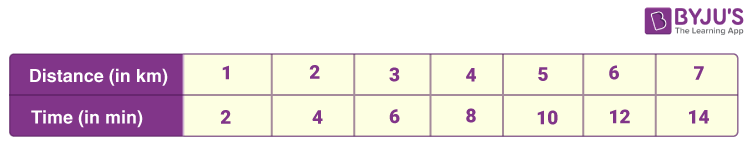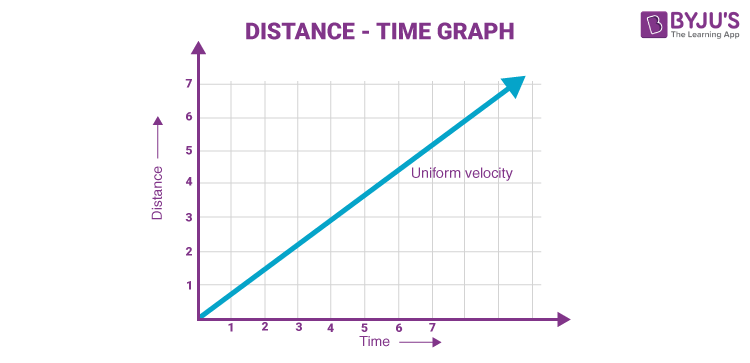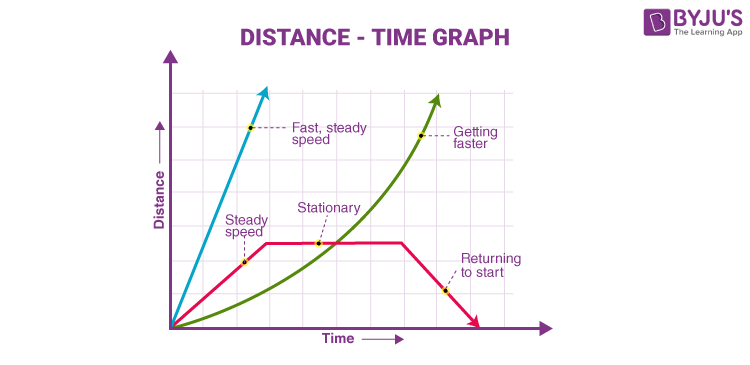## What Is a Distance-Time Graph?

A distance-time graph shows how far an object has travelled in a given time.  It is a simple line graph that denotes distance versus time findings on the graph.

1. Distance is plotted on the Y-axis.
2. Time is plotted on the X-axis.

Note: Curved lines on a distance-time graph indicate that the speed is changing.

You may also want to check out these topics given below!

## Importance of Distance-Time Graph

We deal with the distance-time graph while studying the motion of bodies. If we record distance and time for the motion of a body and plot the same data on a rectangular graph, we will obtain a distance-time graph corresponding to the motion of that body.

### Example:

For better understanding, let us consider an example of uniform motion. A bus driver drives at a constant speed which is indicated by the speedometer and the driver measures the time taken by the bus for every kilometre. The driver notices that the bus travels 1 kilometre every 2 minutes.Data Table

He prepares the data table after this so that he has a clear understanding of everything and then draws the graph as shown below.By this table, he had a clear idea about the speed which is: ½ × 60 = 30 km/hr.

The graph is a straight line and the motion of the bus is also uniform. Also, from the graph, we can find the speed of the bus at any instant of time. The initial and final position of the car can be found as the following:

Speed = (Final Position-Initial position)/Time

The slope of the line can be found by drawing a rectangle anywhere near the straight line which determines the speed of the bus. If an object is not moving, the distance-time graph results in a horizontal line which shows that the object is at rest.## Conclusion:

The following things can be concluded now:

• If the distance-time graph is a straight line then the motion is uniform.
• If the distance-time graph of a body is given, its speed can be calculated using the slope of the graph.
• The slope of the straight-line graph is the same irrespective of the interval which is chosen. This implies that the speed of an object under uniform motion remains constant.

## What is Velocity -Time Graph and Displacement-Time Graph?## Frequently Asked Questions – FAQs

Q1

### What is a graph?

A graph is defined as a pictorial representation of information which is a two-dimensional drawing showing the relationship between dependent and independent variables. Independent variables are denoted on the horizontal line known as the x-axis, while the dependent variables are denoted on the vertical line known as the y-axis.
Q2

### What are the main components of a 2D graph?

The main components of a 2D graph are x-coordinate and y-coordinate.
Q3

### What are the three common types of graphs in classical mechanics?

Displacement time graph, velocity-time graph, and acceleration time graph are three common types of graphs in classical mechanics.
Q4

### What is the distance-time graph?

In the distance-time graph, distance is the dependent variable and is represented on the y-axis, while time is the independent variable and is represented on the x-axis.
Q5

### What does the slope of the distance-time graph represent?

The slope of the distance-time graph represents the speed of an object.

Stay tuned to BYJU’S and Fall in Love with Learning!

Test your knowledge on Distance-time graph

1. excellent answer i have got

3. Thank you so much

4. Very clearly ..I understand easily .. GREAT 👍👍👍

5. u did really well . your efforts wont go unnoticed . u rock!

• Yes they are really great

6. Thank you BYJU’S

7. Tthank you great and clear

8. It is really amazing I understand it thank you byju’s

9. Really, that was excellent.

10. I have a got a great answer thanks